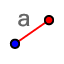# Triangle Congruence: HL

## Construct a right triangle with a hypotenuse of length 17 and a leg of length 8.

1. Select the SEGMENT WITH GIVEN LENGTHtool, and enter a length of 8. 2. Select the PERPENDICULAR LINEtool. Click on segment AB and then on point A. 3. Select the CIRCLE: CENTER & RADIUStool. Click points B, and enter a radius of 17. 4. Use the INTERSECTtool to mark the third vertex of your circle as point C.

Construct a right triangle with a hypotenuse of length 17 and a leg of length 8. What are the other two angles? What is the third side? (Confirm the third side with the Pythagorean Theorem).

Construct a right triangle with a hypotenuse of length 13 and a leg of length 5. What are the other two angles? What is the third side? (Confirm the third side with the Pythagorean Theorem).